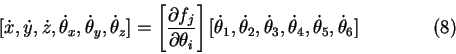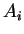Next: Iterative model Up: Iterative methods Previous: Jacobian inversion method

### Jacobian construction

If the analytic expression is known for equation (1), then the evaluation of the Jacobian could be done by straightforward differentiation. For example, equation (6) for a structure with 6 DOF in 6D Cartesian space (3D for position, 3D for rotation) - (Figure 2b) can be expressed as:If the analytic expression is unknown for equation (1), numerical construction for the Jacobian is used. The Jacobian is obtained column by column from the transformation matrices[Chi96].

Lukas Barinka 2002-03-21